Oxidation and Reduction

Oxidation and Reduction

The term oxidation was originally used to describe reactions in which an element combines with oxygen.

Example: The reaction between magnesium metal and oxygen to form magnesium oxide involves the oxidation of magnesium.The term reduction comes from the Latin stem meaning "to lead back." Anything that that leads back to magnesium metal therefore involves reduction.

The reaction between magnesium oxide and carbon at 2000C to form magnesium metal and carbon monoxide is an example of the reduction of magnesium oxide to magnesium metal.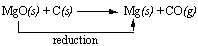After electrons were discovered, chemists became convinced that oxidation-reduction reactions involved the transfer of electrons from one atom to another. From this perspective, the reaction between magnesium and oxygen is written as follows.

2 Mg + O22 [Mg2+][O2-]

In the course of this reaction, each magnesium atom loses two electrons to form an Mg2+ ion.

MgMg2+ + 2 e-

And, each O2 molecule gains four electrons to form a pair of O2- ions.

O2 + 4 e-2 O2-

Because electrons are neither created nor destroyed in a chemical reaction, oxidation and reduction are linked. It is impossible to have one without the other, as shown in the figure below.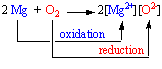Practice Problem 1:Determine which element is oxidized and which is reduced when lithium reacts with nitrogen to form lithium nitride. 6 Li(s) + N2(g)2 Li3N(s) Click here to check your answer to Practice Problem 1Chemists eventually extended the idea of oxidation and reduction to reactions that do not formally involve the transfer of electrons.

Consider the following reaction.

CO(g) + H2O(g)CO2(g) + H2(g)

As can be seen in the figure below, the total number of electrons in the valence shell of each atom remains constant in this reaction.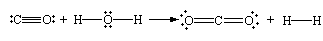What changes in this reaction is the oxidation state of these atoms. The oxidation state of carbon increases from +2 to +4, while the oxidation state of the hydrogen decreases from +1 to 0.Oxidation and reduction are therefore best defined as follows. Oxidation occurs when the oxidation number of an atom becomes larger. Reduction occurs when the oxidation number of an atom becomes smaller.

 Practice Problem 2:Determine which atom is oxidized and which is reduced in the following reaction Sr(s) + 2 H2O(l)Sr2+(aq) + 2 OH-(aq) + H2(g) Click here to check your answer to Practice Problem 2Oxidation Numbers Versus the True Charge on Ions

The terms ionic and covalent describe the extremes of a continuum of bonding. There is some covalent character in even the most ionic compounds and vice versa.

It is useful to think about the compounds of the main group metals as if they contained positive and negative ions. The chemistry of magnesium oxide, for example, is easy to understand if we assume that MgO contains Mg2+ and O2- ions. But no compounds are 100% ionic. There is experimental evidence, for example, that the true charge on the magnesium and oxygen atoms in MgO is +1.5 and -1.5.

Oxidation states provide a compromise between a powerful model of oxidation-reduction reactions based on the assumption that these compounds contain ions and our knowledge that the true charge on the ions in these compounds is not as large as this model predicts. By definition, the oxidation state of an atom is the charge that atom would carry if the compound were purely ionic.

For the active metals in Groups IA and IIA, the difference between the oxidation state of the metal atom and the charge on this atom is small enough to be ignored. The main group metals in Groups IIIA and IVA, however, form compounds that have a significant amount of covalent character. It is misleading, for example, to assume that aluminum bromide contains Al3+ and Br- ions. It actually exists as Al2Br6 molecules.

This problem becomes even more severe when we turn to the chemistry of the transition metals. MnO, for example, is ionic enough to be considered a salt that contains Mn2+ and O2- ions. Mn2O7, on the other hand, is a covalent compound that boils at room temperature. It is therefore more useful to think about this compound as if it contained manganese in a +7 oxidation state, not Mn7+ ions.Oxidizing Agents and Reducing Agents

Let's consider the role that each element plays in the reaction in which a particular element gains or loses electrons..

When magnesium reacts with oxygen, the magnesium atoms donate electrons to O2 molecules and thereby reduce the oxygen. Magnesium therefore acts as a reducing agent in this reaction.

 2 Mg + O22 MgO reducing agent

The O2 molecules, on the other hand, gain electrons from magnesium atoms and thereby oxidize the magnesium. Oxygen is therefore an oxidizing agent.

 2 Mg + O22 MgO oxidizing agent

Oxidizing and reducing agents therefore can be defined as follows. Oxidizing agents gain electrons. Reducing agents lose electrons.

 Practice Problem 3:Identify the oxidizing agent and the reducing agent in the following reaction. Ca(s) + H2(g)CaH2(g) Click here to check your answer to Practice Problem 3

The table below identifies the reducing agent and the oxidizing agent for some of the reactions discussed in this web page. One trend is immediately obvious: The main group metals act as reducing agents in all of their chemical reactions.

Typical Reactions of Main Group Metals

 Reaction Reducing Agent Oxidizing Agent 2 Na + Cl22 NaCl Na Cl2 2 K + H22 KH K H2 4 Li + O22 Li2O Li O2 2 Na + O2Na2O2 Na O2 2 Na + 2 H2O2 Na+ + 2 OH- + H2 Na H2O 2 K + 2 NH32 K+ + 2 NH2- + H2 K NH3 2 Mg + O22 MgO Mg O2 3 Mg + N2Mg3N2 Mg N2 Ca + 2 H2OCa2+ + 2 OH- + H2 Ca H2O 2 Al + 3 Br2Al2Br6 Al Br2 Mg + 2 H+Mg2++ H2 Mg H+ Mg + H2OMgO + H2 Mg H2OMetals act as reducing agents in their chemical reactions. When copper is heated over a flame, for example, the surface slowly turns black as the copper metal reduces oxygen in the atmosphere to form copper(II) oxide.If we turn off the flame, and blow H2 gas over the hot metal surface, the black CuO that formed on the surface of the metal is slowly converted back to copper metal. In the course of this reaction, CuO is reduced to copper metal. Thus, H2 is the reducing agent in this reaction, and CuO acts as an oxidizing agent.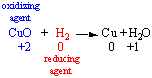An important feature of oxidation-reduction reactions can be recognized by examining what happens to the copper in this pair of reactions. The first reaction converts copper metal into CuO, thereby transforming a reducing agent (Cu) into an oxidizing agent (CuO). The second reaction converts an oxidizing agent (CuO) into a reducing agent (Cu). Every reducing agent is therefore linked, or coupled, to a conjugate oxidizing agent, and vice versa.

Every time a reducing agent loses electrons, it forms an oxidizing agent that could gain electrons if the reaction were reversed.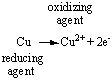Conversely, every time an oxidizing agent gains electrons, it forms a reducing agent that could lose electrons if the reaction went in the opposite direction.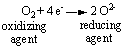The idea that oxidizing agents and reducing agents are linked, or coupled, is why they are called conjugate oxidizing agents and reducing agents. Conjugate comes from the Latin stem meaning "to join together." It is therefore used to describe things that are linked or coupled, such as oxidizing agents and reducing agents.

The main group metals are all reducing agents. They tend to be "strong" reducing agents. The active metals in Group IA, for example, give up electrons better than any other elements in the periodic table.

The fact that an active metal such as sodium is a strong reducing agent should tell us something about the relative strength of the Na+ ion as an oxidizing agent. If sodium metal is relatively good at giving up electrons, Na+ ions must be unusually bad at picking up electrons. If Na is a strong reducing agent, the Na+ ion must be a weak oxidizing agent.Conversely, if O2 has such a high affinity for electrons that it is unusually good at accepting them from other elements, it should be able to hang onto these electrons once it picks them up. In other words, if O2 is a strong oxidizing agent, then the O2- ion must be a weak reducing agent.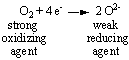In general, the relationship between conjugate oxidizing and reducing agents can be described as follows. Every strong reducing agent (such as Na) has a weak conjugate oxidizing agent (such as the Na+ ion). Every strong oxidizing agent (such as O2) has a weak conjugate reducing agent (such as the O2- ion).The Relative Strength of Metals as Reducing Agents

We can determine the relative strengths of a pair of metals as reducing agents by determining whether a reaction occurs when one of these metals is mixed with a salt of the other. Consider the relative strength of iron and aluminum, for example. Nothing happens when we mix powdered aluminum metal with iron(III) oxide. If we place this mixture in a crucible, however, and get the reaction started by applying a little heat, a vigorous reaction takes place to give aluminum oxide and molten iron metal.

2 Al(s) + Fe2O3(s)Al2O3(s) + 2 Fe(l)

By assigning oxidation numbers, we can pick out the oxidation and reduction halves of the reaction.Aluminum is oxidized to Al2O3 in this reaction, which means that Fe2O3 must be the oxidizing agent. Conversely, Fe2O3 is reduced to iron metal, which means that aluminum must be the reducing agent. Because a reducing agent is always transformed into its conjugate oxidizing agent in an oxidation-reduction reaction, the products of this reaction include a new oxidizing agent (Al2O3) and a new reducing agent (Fe).Since the reaction proceeds in this direction, it seems reasonable to assume that the starting materials contain the stronger reducing agent and the stronger oxidizing agent.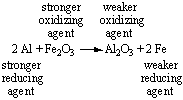In other words, if aluminum reduces Fe2O3 to form Al2O3 and iron metal, aluminum must be a stronger reducing agent than iron.

We can conclude from the fact that aluminum cannot reduce sodium chloride to form sodium metal that the starting materials in this reaction are the weaker oxidizing agent and the weaker reducing agent.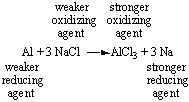We can test this hypothesis by asking: What happens when we try to run the reaction in the opposite direction? (Is sodium metal strong enough to reduce a salt of aluminum to aluminum metal?) When this reaction is run, we find that sodium metal can, in fact, reduce aluminum chloride to aluminum metal and sodium chloride when the reaction is run at temperatures hot enough to melt the reactants.

3 Na(l) + AlCl3(l)3 NaCl(l) + Al(l)

If sodium is strong enough to reduce Al3+ salts to aluminum metal and aluminum is strong enough to reduce Fe3+ salts to iron metal, the relative strengths of these reducing agents can be summarized as follows.

Na > Al > Fe

Practice Problem 4:

Use the following equations to determine the relative strengths of sodium, magnesium, aluminum, and calcium metal as reducing agents.

2 Na + MgCl22 NaCl + Mg
 Al + MgBr2Ca + MgI2CaI2 + Mg
 Ca + 2 NaCl